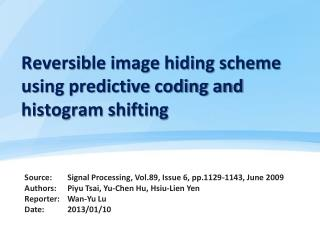DownloadDownload PresentationReversible image hiding scheme using predictive coding and histogram shifting

# Reversible image hiding scheme using predictive coding and histogram shifting

Télécharger la présentation## Reversible image hiding scheme using predictive coding and histogram shifting

- - - - - - - - - - - - - - - - - - - - - - - - - - - E N D - - - - - - - - - - - - - - - - - - - - - - - - - - -
##### Presentation Transcript

1. Reversible image hiding scheme using predictive coding and histogram shifting

2. Outline • Introduction • Related work- Ni et al.s scheme (The histogram-based reversible data hiding ) • The proposed method- Embedding phase - Extraction phase • Experimental results • Conclusion • Comparatives

3. Introduction Embedding algorithm stego-image Secret data Original image Embedded image Extraction algorithm

4. Related work (1/2) Peak = 3 Zero = 7 peak Zero Originalimage pixels Ni, Zhicheng., Shi, Y.Q., Ansari, N. and Su, Wei. (2006), Reversible Data Hiding, IEEE Transactions on Circuits and Systems for Video Technology,16 (3), pp. 354-362.

5. Related work (2/2) Secret data = 1 0 1 1 0 0 Embedded image pixels Ni, Zhicheng., Shi, Y.Q., Ansari, N. and Su, Wei. (2006), Reversible Data Hiding, IEEE Transactions on Circuits and Systems for Video Technology,16 (3), pp. 354-362.

6. The proposed method (1/6) • Embedding phase error value = basic pixel - residual pixel Originalimage

7. The proposed method (2/6) • Embedding phase peak2 peak1 zero1 zero2 peak1 = -1, zero1 = -4 peak2 = 0, zero2 = 4

8. The proposed method (3/6) • Embedding phase Secret data={ 110000010010100110}

9. The proposed method (4/6) • Embedding phase residual pixel = basic pixel - error value Embedded image

10. The proposed method (5/6) peak1 = -1, zero1 = -4 peak2 = 0, zero2 = 4 • Extraction phase Secret data={ 110000010010100110}

11. The proposed method (6/6) • Extraction phase

12. Experimental results (1/4)

13. Experimental results (3/4)

14. Experimental results (4/4) Ni et al’s scheme peak=16591 Capacity=16591 bits Proposed scheme peak=23723 peak=13539 Capacity=37262 bits

15. Conclusion To achieve a higher hiding capacity , secret data are embedded in the residual images instead of the image histogram.

16. Comparisons • To compare with related work: • - Reversible medical image watermarking based onwavelet histogram shifting (2011) • Same • - Reversible medical image hiding based onhistogram shifting. • - Two-pairs • Difference • - spatial domain vs. transformed domain (IDWT) • - overflow and underflow